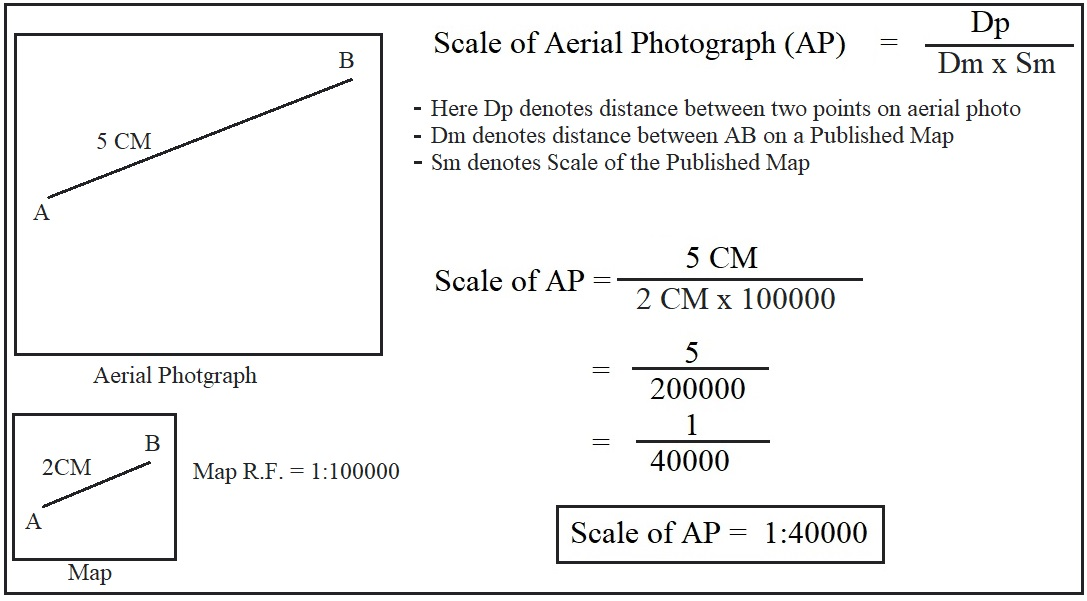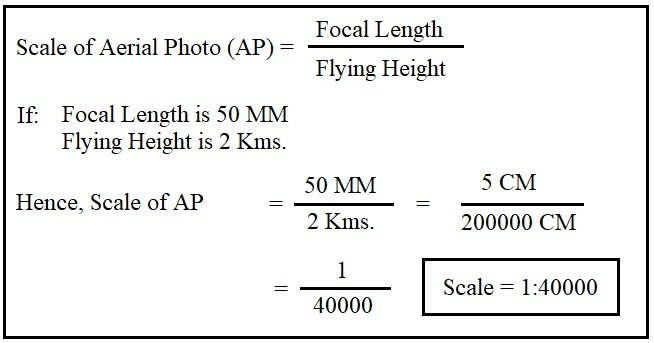# Scale of Aerial Photography

Scale of aerial photography refers to the proportion of distance between two points on the aerial photograph and the distance between the same points on the ground. It is necessary to know the scale of an aerial photograph for the interpretation and photogrammetry. There are three scenarios where we need to calculate the scale. We can use the following methods of calculating scale of aerial photographs in these scenarios.

## 1. By Using Ground Distance

• We can calculate the scale of an aerial photograph when we know the actual or ground distance between two points on the aerial photograph.
• Assume that the distance between two points on an aerial photograph is 5 CM and the actual distance between the same points on ground is 2 Kms. In this case, the scale of the aerial photo will be as per the following calculations.
• Here Dp denotes Distance between two points on Aerial Photo and Dg refers to distance between the same two points on ground.

## 2. By Using a Published Map

• In this method, the surveyor knows the distance between two points on the aerial photograph (Dp) but does not know the actual distance between two points on the ground (Dg).
• In this case, we can use an available map of the area under survey.
• Assume that we are surveying the Central Vista in Delhi and the distance between India gate and Rashtrapati Bhawan (president’s residence) is 5 CM on an aerial photograph. However, we do not know the actual distance between these two locations.
• Then we will take an already published map or Topographic Sheet of Central Vista and calculate the scale using the following formulae.Fig. 2: Formulae to Calculate Scale of Aerial Photo with the help of a Map
• In Fig. 2, the distance between A-B is 5 CM on aerial photo. The distance between A-B is 2 CM on a published map and the scale or R.F. is 1: 100000.
• We can apply the given formulae and arrive at the scale of the aerial photo as shown in Fig. 2.

## 3. By Using the Focal Length

• Focal length refers to the distance between the lens and the film plane. For details, read Geometry of Aerial Photography.
• Usually, we determine the scale of an aerial photograph before starting the survey.
• We can do so by using the focal length of the camera and the flying height as follows.Fig. 3: Calculation of Scale by Using Focal Length
• Assume that the focal length of the camera is 50 MM and the flying height of the airplane is 2 Kms. In this case, we will calculate the scale of the aerial photo as follows.

## Conclusion

In summary, we can argue that there are multiple ways of calculating the scale of aerial photographs. These methods include the use of ground distance, published maps and focal length. Usually, a surveyor chooses a lens with a certain focal length, thereafter, he chooses the flying height for capturing photos. These two variables determine the scale of aerial photos. Nonetheless, other methods can be used in case the data pertaining focal length and flying height is lost or not available.